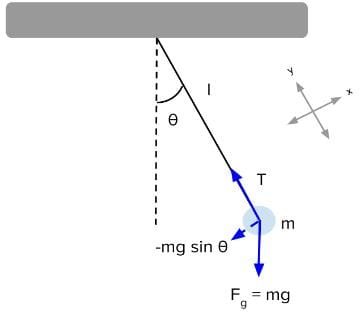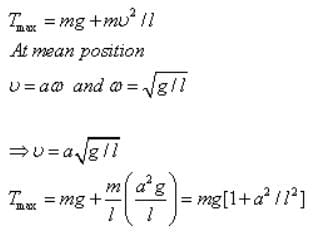NEET  >  Test: Illustrations of Bodies in Simple Harmonic Motion

# Test: Illustrations of Bodies in Simple Harmonic Motion

Test Description

## 10 Questions MCQ Test Physics Class 11 | Test: Illustrations of Bodies in Simple Harmonic Motion

Test: Illustrations of Bodies in Simple Harmonic Motion for NEET 2023 is part of Physics Class 11 preparation. The Test: Illustrations of Bodies in Simple Harmonic Motion questions and answers have been prepared according to the NEET exam syllabus.The Test: Illustrations of Bodies in Simple Harmonic Motion MCQs are made for NEET 2023 Exam. Find important definitions, questions, notes, meanings, examples, exercises, MCQs and online tests for Test: Illustrations of Bodies in Simple Harmonic Motion below.
Solutions of Test: Illustrations of Bodies in Simple Harmonic Motion questions in English are available as part of our Physics Class 11 for NEET & Test: Illustrations of Bodies in Simple Harmonic Motion solutions in Hindi for Physics Class 11 course. Download more important topics, notes, lectures and mock test series for NEET Exam by signing up for free. Attempt Test: Illustrations of Bodies in Simple Harmonic Motion | 10 questions in 10 minutes | Mock test for NEET preparation | Free important questions MCQ to study Physics Class 11 for NEET Exam | Download free PDF with solutions
 1 Crore+ students have signed up on EduRev. Have you?
Test: Illustrations of Bodies in Simple Harmonic Motion - Question 1

### Two point masses of 3 Kg and 2 Kg are attached at the two ends of a horizontal spring with spring constant k=200N/m. Find the natural frequency of vibration of the system.

Detailed Solution for Test: Illustrations of Bodies in Simple Harmonic Motion - Question 1

The reduced mass of the system
M0=m1 x m2/ m1 + m2=3x2/3+2=6/5 Kg
Inertia factor=6/5
Spring constant, k=200Nm-1
Frequency u=1/2π√spring factor/inertia factor
=1/2π√200/6/5
=2.05Hz

Test: Illustrations of Bodies in Simple Harmonic Motion - Question 2

### The instantaneous displacement of a simple harmonic oscillator is given by y = A cos (ωt + n/4). Its speed will be maximum at the time:). Its speed will be maximum at the time:

Detailed Solution for Test: Illustrations of Bodies in Simple Harmonic Motion - Question 2

The instantaneous displacement of a simple pendulum oscillator is given as y = acos(ωt + π/4)

differentiating with respect to time,
dy/dt = -ωasin(ωt + π/4)
here, dy/dt is the velocity of a particle executing SHM.
so, speed of particle = | dy/dt | = ωasin(ωt + π/4)
so, dy/dt will be maximum when sin(ωt + π/4) will be maximum i.e., 1
so, sin(ωt + π/4) = 1 = sin(π/2)
⇒ωt + π/4 = π/2
⇒ωt = π/4
⇒t = π/(4ω)
hence, at t = π/(4ω) , speed of the particle will be maximum.

Test: Illustrations of Bodies in Simple Harmonic Motion - Question 3

### Due to what force a simple pendulum remains in simple harmonic motion?

Detailed Solution for Test: Illustrations of Bodies in Simple Harmonic Motion - Question 3

For the pendulum, we can use Newton's second law to write an equation for the forces on the pendulum. The only force responsible for the oscillating motion of the pendulum is the x-component of the weight, so the restoring force on a pendulum is:
F=−mg sinθ
For angles under about 15°, we can approximate sinθ as θ and the restoring force simplifies to:
F ≈ −mgθ
Thus, simple pendulums are simple harmonic oscillators for small displacement angles.Test: Illustrations of Bodies in Simple Harmonic Motion - Question 4

The motion of simple pendulum is said to be S.H.M when its angle Î¸Â¸ through which bob is displaced from its equilibrium position is

Detailed Solution for Test: Illustrations of Bodies in Simple Harmonic Motion - Question 4

When angle is very small then the value of sin of angle becomes almost 1. This is the one of the necessary conditions for SHM

Test: Illustrations of Bodies in Simple Harmonic Motion - Question 5

Two light springs of force constants k1 and k2, loaded with equal masses undergo vertical oscillations. If the maximum velocities of the masses are the same, the ratio of the amplitudes of oscillations is:

Detailed Solution for Test: Illustrations of Bodies in Simple Harmonic Motion - Question 5

As the maximum velocities are equal
a1ω1 = a2ω2
a1/a2 = ω2/ω1 ----- (1)
as, k1 = mω12 ----- (2)
k2 = mω22 ----- (3)
substituting eq 2 and eq 3 in eq 1 ,we get
a1/a2 = (k1/k2)1/2

Test: Illustrations of Bodies in Simple Harmonic Motion - Question 6

The work done by the string of a simple pendulum in S.H.M is

Test: Illustrations of Bodies in Simple Harmonic Motion - Question 7

A simple pendulum with length l and bob of mass m is executing S.H.M of small amplitude a. The expression for maximum tension in the string will be

Detailed Solution for Test: Illustrations of Bodies in Simple Harmonic Motion - Question 7

The tension in the string would be maximum when bob will be passing mean position.Test: Illustrations of Bodies in Simple Harmonic Motion - Question 8

The amplitude A of a simple harmonic oscillator (with period, T and energy, E) is tripled. What would happen to T and E?

Detailed Solution for Test: Illustrations of Bodies in Simple Harmonic Motion - Question 8

E α A2 and hence the energy will become 9 times.
T is independent of A and hence no change will occur.
Hence C is the correct answer.

Test: Illustrations of Bodies in Simple Harmonic Motion - Question 9

A simple pendulum is arranged using a small metallic bob of mass ‘m’and a light rubber cord of length ‘L’ (on suspending the bob), area of cross section ‘A’ and Young’s modulus ‘Y’. [One should use inextensible cord only for simple pendulum!]. When this unconventional pendulum is at rest in its mean position, the bob is pulled slightly down and is released. Then, the period of the vertical oscillation of the bob is (assuming that the size of the bob is negligible compared to the length of the cord):

Detailed Solution for Test: Illustrations of Bodies in Simple Harmonic Motion - Question 9

The period as usual is given by T = 2n√(m/k). Here `m' is the same as the mass of the bob. The force constant can be found by writing the expression for Young's modulus (since it arises from the elastic force in the cord): V = FL/A(δL) where 6L is the increase in the length of the cord on pulling the bob down with a force F. Therefore, the force constant, F/(δL) = YA/L. On substituting this value, the period is 2m✓(mL/YA).

Test: Illustrations of Bodies in Simple Harmonic Motion - Question 10

A body of mass 5.0 kg is suspended by a spring which stretches 10 cm when the mass is attached. It is then displaced downward an additional 5.0 cm and released. Its position as a function of time is approximately:

## Physics Class 11

127 videos|464 docs|210 tests
 Use Code STAYHOME200 and get INR 200 additional OFF Use Coupon Code
Information about Test: Illustrations of Bodies in Simple Harmonic Motion Page
In this test you can find the Exam questions for Test: Illustrations of Bodies in Simple Harmonic Motion solved & explained in the simplest way possible. Besides giving Questions and answers for Test: Illustrations of Bodies in Simple Harmonic Motion, EduRev gives you an ample number of Online tests for practice

## Physics Class 11

127 videos|464 docs|210 tests

### How to Prepare for NEET

Read our guide to prepare for NEET which is created by Toppers & the best Teachers